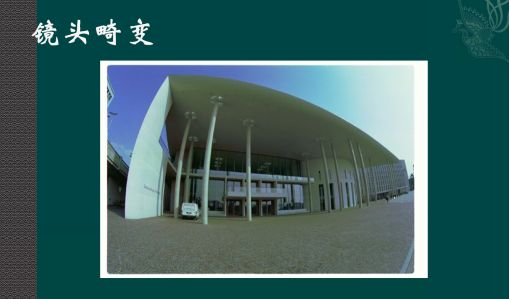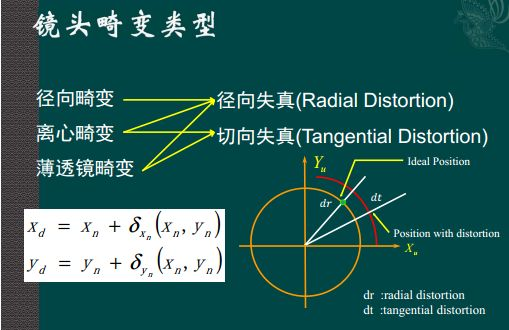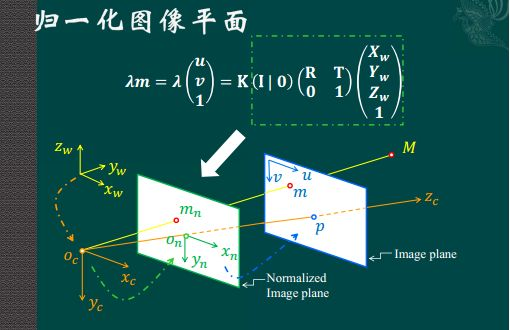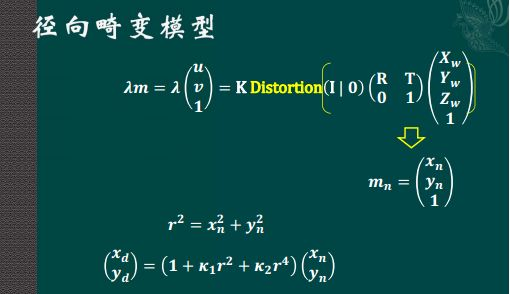## 摄像机成像的几何模型

2015年03月27日

### 投影过程一览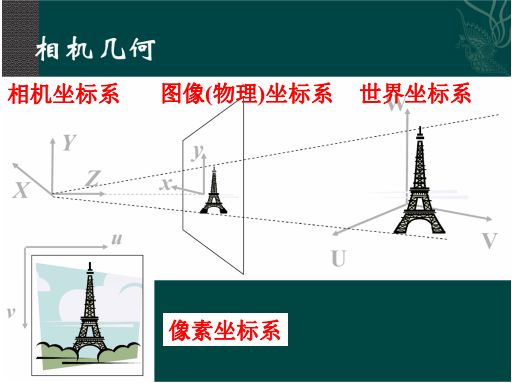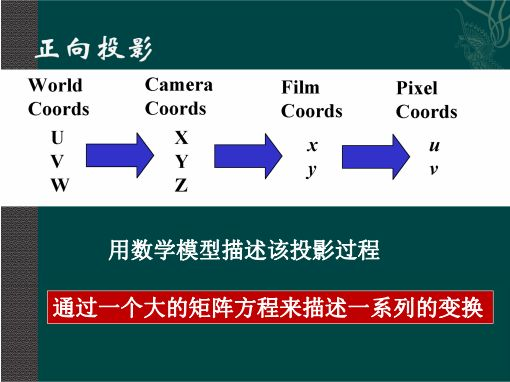### 世界坐标系到相机坐标系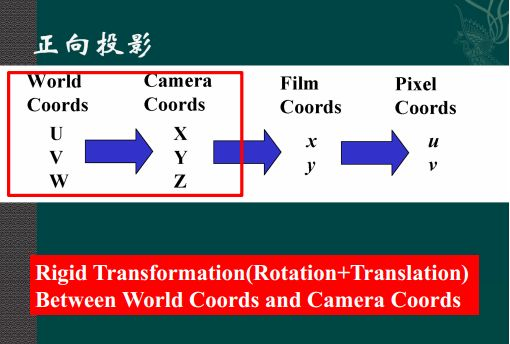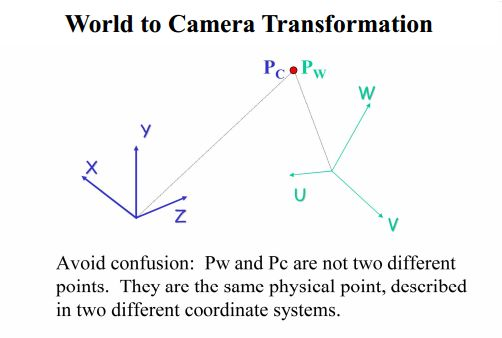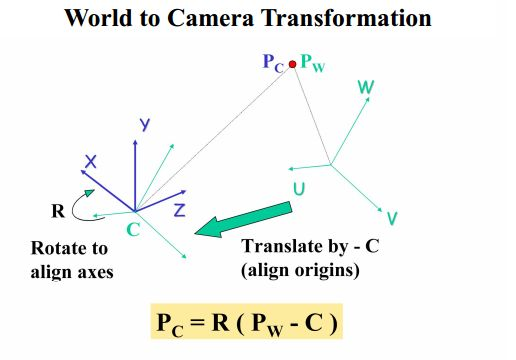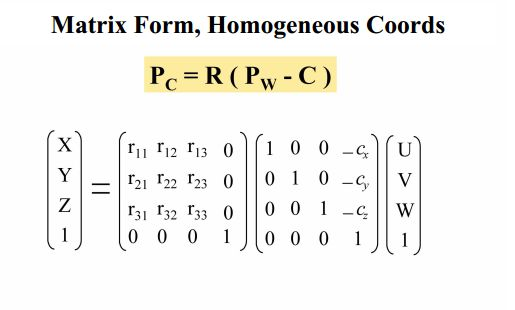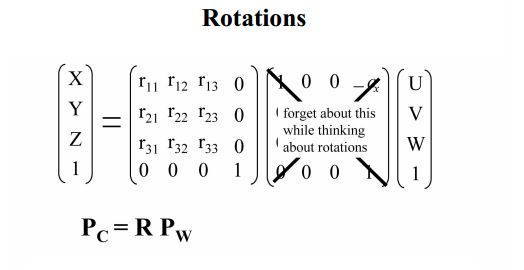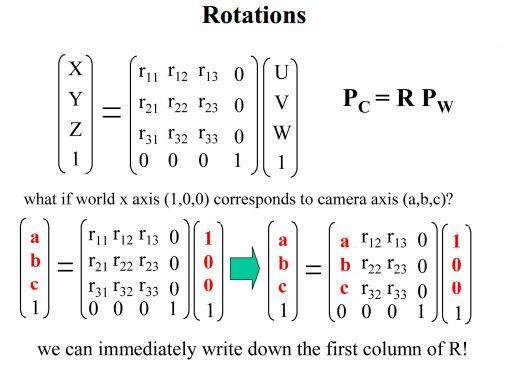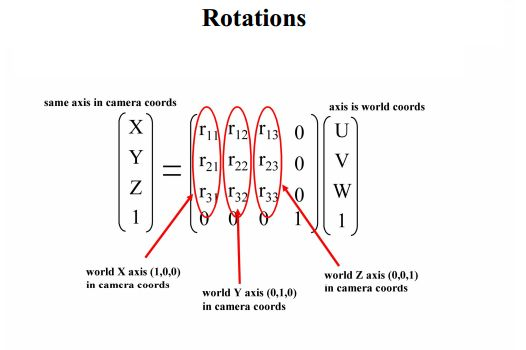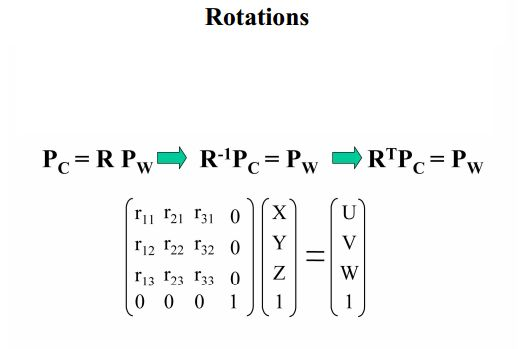### 相机坐标系到图像（物理）坐标系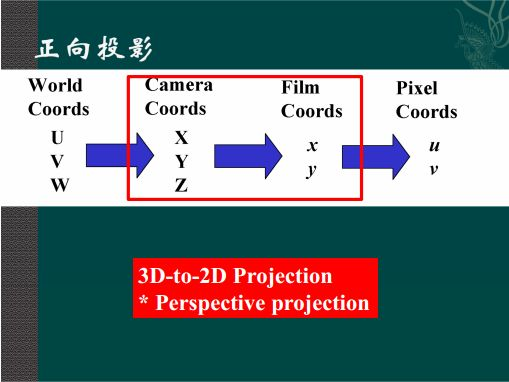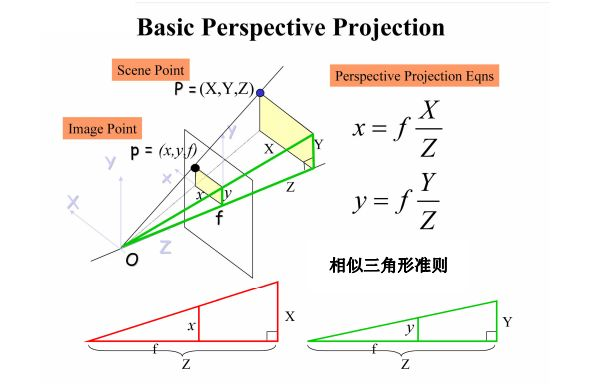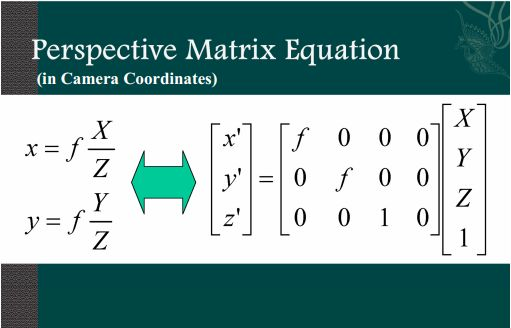### 图像（物理）坐标系到像素坐标系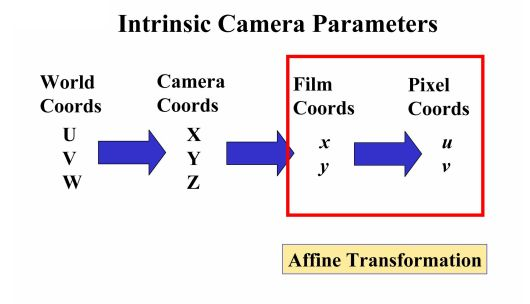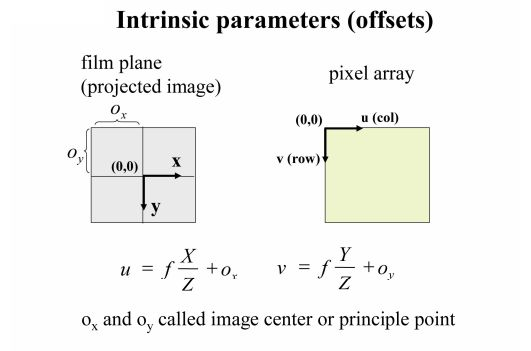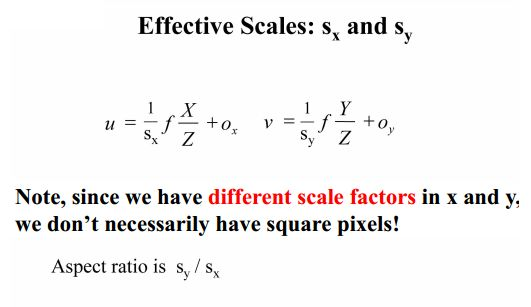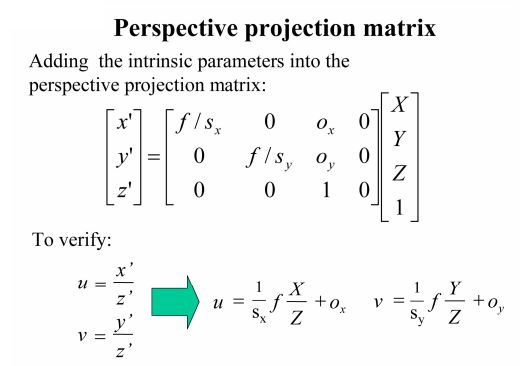### 坐标系转换总览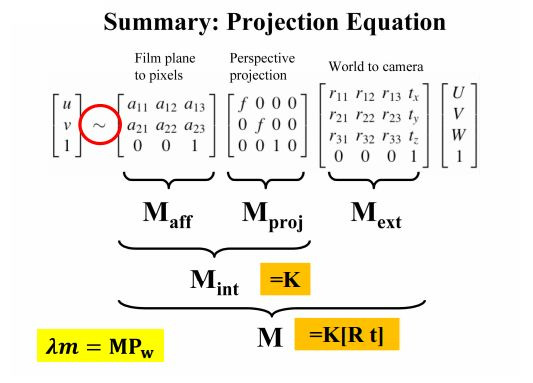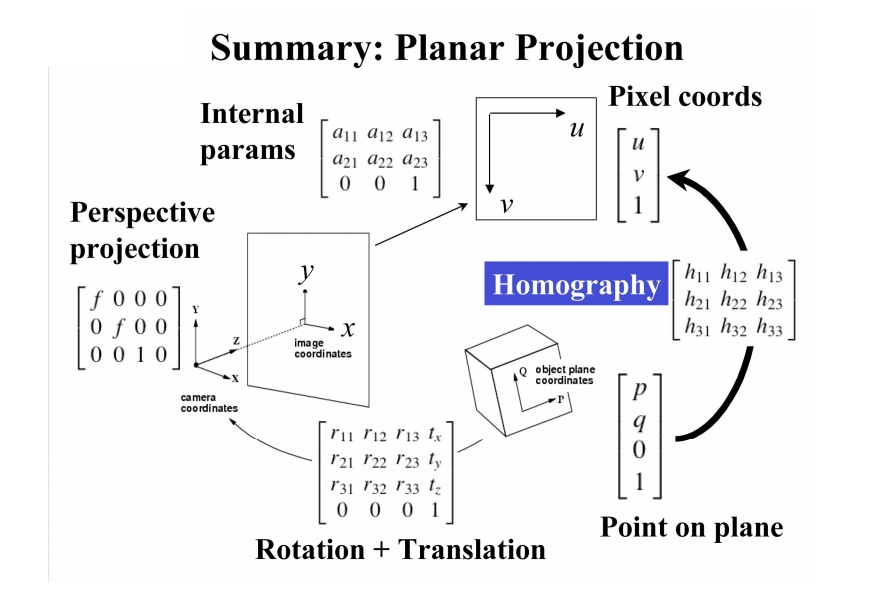\begin{equation} \mathbb{K}=\begin{bmatrix} \alpha & -\alpha \cot\theta & o_{x} \\ 0 & \frac{\beta}{\sin\theta} & o_{y} \\ 0 & 0 & 1 \end{bmatrix} \end{equation}

\begin{equation} \mathbb{M}=\mathbb{K}[\mathbb{R} \;\, \mathbf{t}]=\begin{bmatrix} \alpha\mathbf{r}_{1}^{T}-\alpha\cot\theta\mathbf{r}_{2}^{T}+o_{x}\mathbf{r}_{3}^{T} & \alpha t_{x} -\alpha\cot\theta t_{y}+o_{x}t_{z} \\ \frac{\beta}{\sin\theta}\mathbf{r}_{2}^{T}+o_{y}\mathbf{r}_{3}^{T} & \frac{\beta}{\sin\theta}t_{y}+o_{y}t_{z} \\ \mathbf{r}_{3}^{T} & t_{z} \end{bmatrix} \end{equation}

### 镜头畸变